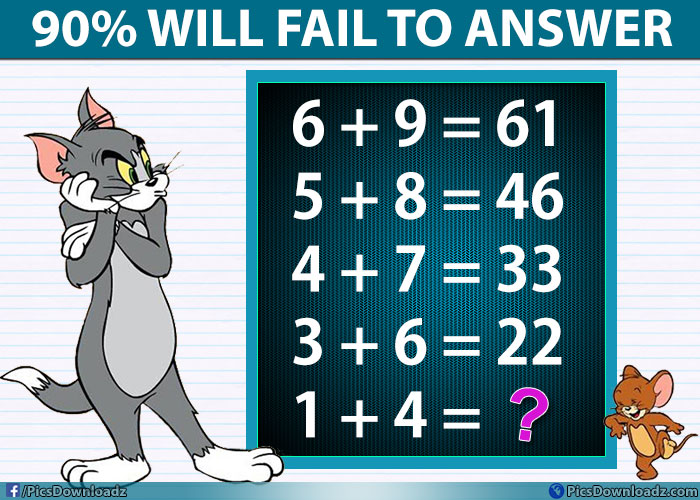This post may contain affiliate links. This means if you click on the link and purchase the item, We will receive an affiliate commission at no extra cost to you. See Our Affiliate Policy for more info.

# If 6+9=61 Then 1+4=?? Logic Math Puzzle Only for Genius

5
6601

### Solve this difficult math puzzle image if you are a genius! Logic Math Puzzle Problem Picture with the solution:

A puzzle of the day: Check out this another interesting and tricky math puzzle image. 90% of people will fail to answer this genius puzzle. In this math problem, you will have to find the logic behind this puzzle and solve it, pretty simple! right? Check the question or image below and give it a try;

Puzzle Question:

6 + 9 = 61

5 + 8 = 46

4 + 7 = 33

3 + 6 = 22

1 + 4 = ??

## Logic Math Puzzle Image:Got it? Before you confirm it, share your answer. The comment section is all your’s. You can check the answer at the end of the post.

You can also try our other interesting puzzles:

Find Out ‘Correct Path Ways’? Solve these 4 Tricky Puzzles to Boost your Brain!

What is my relationship to Elisha? Viral Facebook Puzzle Image

Let’s find the solution:

1st Equation:

In first equation i.e. 6 + 9 = 61

If we multiply 6 and 9 we get 54, but as we know our result is 61. So how much numbers we need to get this result. i.e 61 – 54 = 7

So our equation follows the logic is; 6 × 9 + 7 = 61

2nd Equation:

Given equation is 5 + 8 = 46, Let’s try the same logic

5 × 8 + 7 = 47 (which is incorrect), but if we add the number 6 rather than 7, we get the right result.

5 × 8 + 6 = 46

So if we compare the 1st and 2nd equation, We found the logic behind this puzzle i.e:

We have to multiply the given numbers with each other and then add the next number of the first given number.

Or

you can simply follow this formula to find the answer;  X × Y + (X + 1) = Result.

Where X = first number and Y = Second number.

For eg: in 1st Equation; X = 6 and Y = 9; And the solution become: 6 × 9 + (6+1) =  6 × 9 + 7 = 61

So, here is the solution:

6 + 9 = 61    ⇒    6 × 9 + 7 = 61

5 + 8 = 46   ⇒    5 × 8 + 6 = 46

4 + 7 = 33    ⇒   4 × 7 + 5 = 33

3 + 6 = 22    ⇒   3 × 6 + 4 = 22

1 + 4 = ??     ⇒   1 × 4 + 2 = 6 Ans.

Share this puzzle with your friends, let’s see if they can solve this interesting puzzle or not. Don’t forget to connect with us on facebook, like us and get interesting puzzles and funny updates with other interesting stories.

You can also join our facebook group if you are a fan of puzzles: Only for Genius

Enjoy, Take Care!

Try this: Posted by our fan:

Search items:

Math Puzzle Only for Genius, Logic Puzzle Image, Brain Teasers Math Puzzles, Math Problems, Math Puzzles with Answer, Only for Genius Puzzles, Difficult Math Puzzle Question, Hard Puzzle Image, Genius Math Puzzles, Tricky Puzzle Image, Solve Genius Puzzles, Puzzles for Facebook, Puzzles for Whatsapp, Share Puzzle Image, Math Picture Puzzle,

1.Praveen.S.K.

(6*4 = 54) + (6+1 = 7) = 61
(5*8 = 40) + (5+1 = 6) = 46
(4*7 = 28) + (4+1 = 5) = 33
(3*6 = 18) + (3+1 = 4) = 22
(1*4 = 4) + (1+1 = 2) = 6

2.Jude

negative 8

3.Geoff

FOUND A common denominator, the sequence reduces by 2 as you come down
6+9 61
5+8 46 = 15 46+15= 61
4+7 33 = 13 33+15 = 46
3+6 22 = 11 22+11 = 33
missing number 
2+ 5 13 = 7. 13+9 = 22
1+ 4 6 … 5 6+7 = 13
0+ 3. 3
-0+ 2 1
-0+ 1
-0+0
4.Mubeena
5.Jenny park